# Combustion Reactions Combustion is a chemical reaction where

• Slides: 14
Download presentation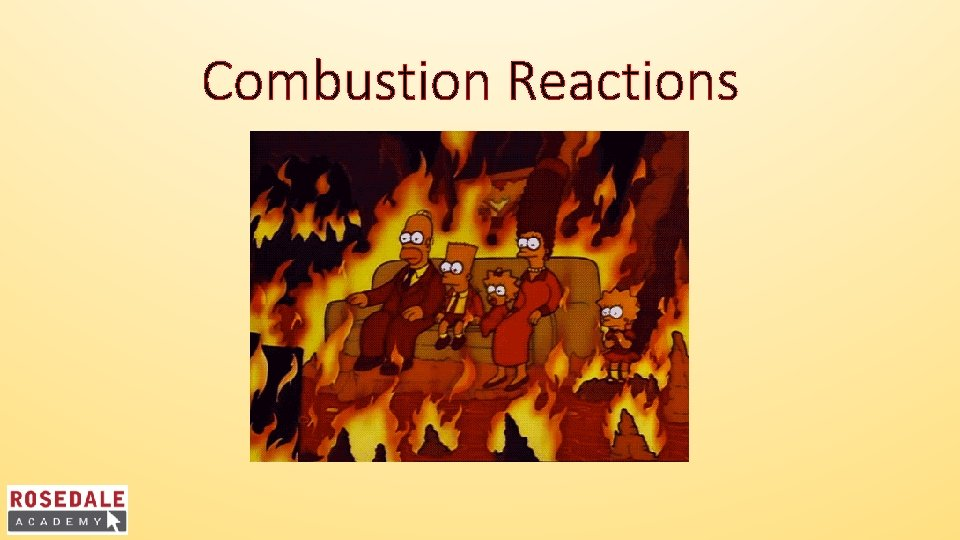Combustion Reactions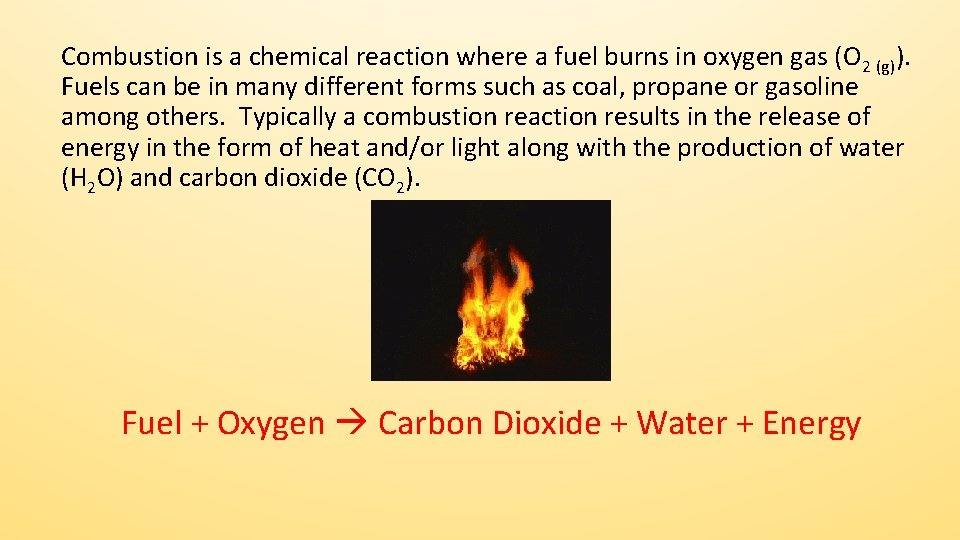Combustion is a chemical reaction where a fuel burns in oxygen gas (O 2 (g)). Fuels can be in many different forms such as coal, propane or gasoline among others. Typically a combustion reaction results in the release of energy in the form of heat and/or light along with the production of water (H 2 O) and carbon dioxide (CO 2). Fuel + Oxygen Carbon Dioxide + Water + Energy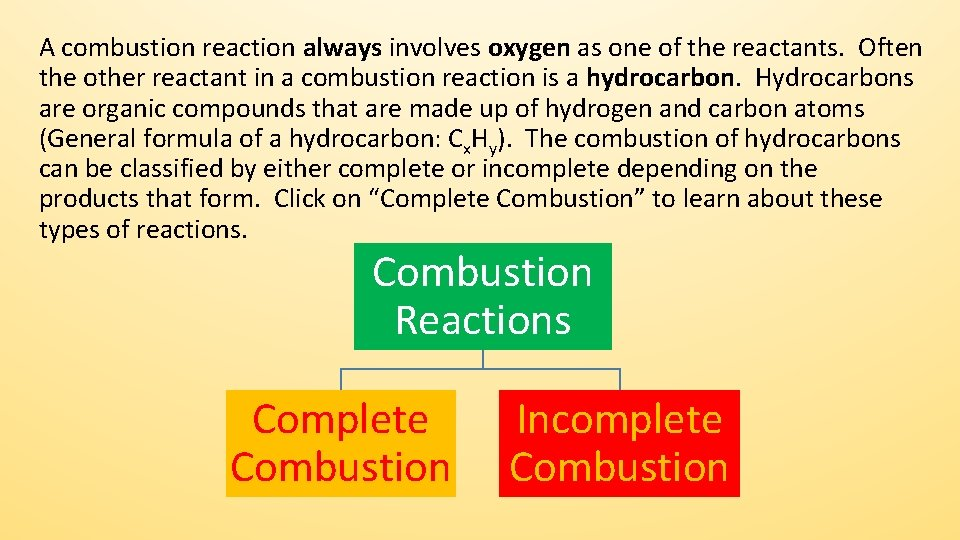A combustion reaction always involves oxygen as one of the reactants. Often the other reactant in a combustion reaction is a hydrocarbon. Hydrocarbons are organic compounds that are made up of hydrogen and carbon atoms (General formula of a hydrocarbon: Cx. Hy). The combustion of hydrocarbons can be classified by either complete or incomplete depending on the products that form. Click on “Complete Combustion” to learn about these types of reactions. Combustion Reactions Complete Combustion Incomplete CombustionComplete Combustion Reactions The complete combustion of a hydrocarbon occurs when a hydrocarbon reacts with oxygen and there is an excess supply of oxygen gas while the reaction proceeds (this means there is more oxygen than needed). The products of a complete combustion reaction are carbon dioxide gas, water vapour and energy. Using this information, write the balanced equation for the complete combustion of methane gas (CH 4). Note: include energy as one of the products). Click for the Balanced Reaction EquationComplete Combustion of Methane The balanced equation for the complete combustion of methane gas is written as: CH 4 (g) + 2 O 2 (g) CO 2 (g) + H 2 O (g) + energy Complete combustion is the ideal way to burn a fuel as is releases the most possible energy from the fuel molecules present. This is also the cleanest way to burn a fuel as it produces the cleanest flames (less soot). Test Your UnderstandingTest Your Understanding Write the balanced chemical equation for the complete combustion of liquid benzene (C 6 H 6): Click for the AnswerTest Your Understanding Answer Write the balanced chemical equation for the complete combustion of liquid benzene (C 6 H 6): 2 C 6 H 6 (l) + 15 O 2 (g) 12 CO 2 (g) + 6 H 2 O (g) + energy Click to Learn About Incomplete Combustion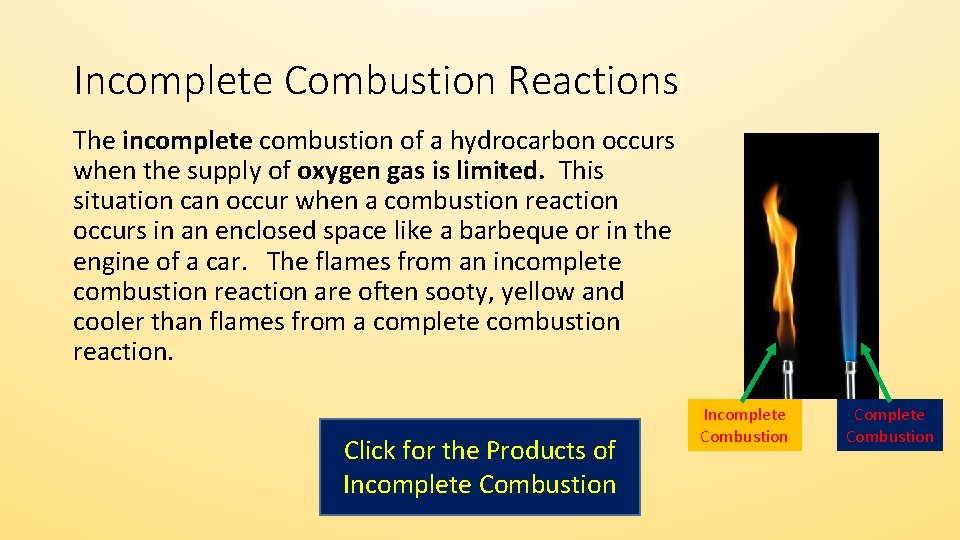Incomplete Combustion Reactions The incomplete combustion of a hydrocarbon occurs when the supply of oxygen gas is limited. This situation can occur when a combustion reaction occurs in an enclosed space like a barbeque or in the engine of a car. The flames from an incomplete combustion reaction are often sooty, yellow and cooler than flames from a complete combustion reaction. Click for the Products of Incomplete Combustion Complete Combustion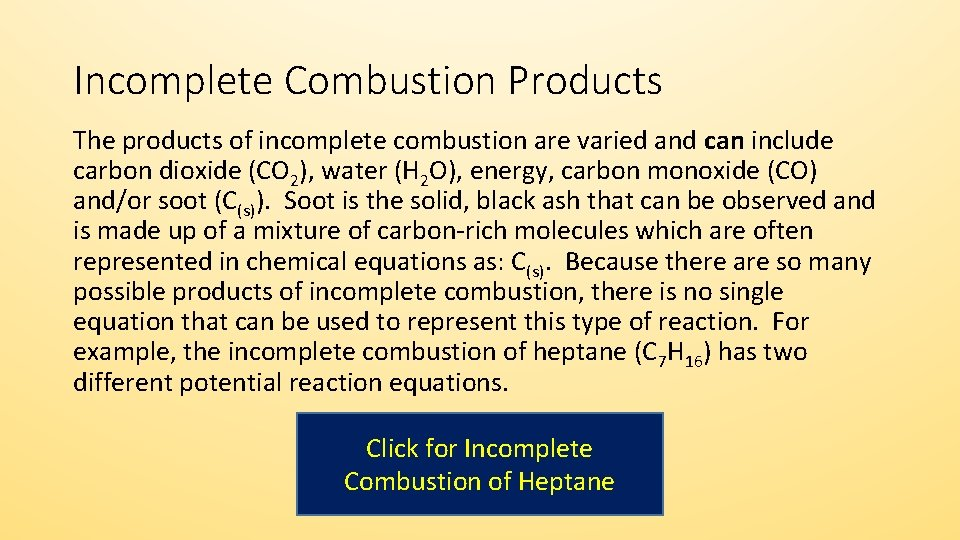Incomplete Combustion Products The products of incomplete combustion are varied and can include carbon dioxide (CO 2), water (H 2 O), energy, carbon monoxide (CO) and/or soot (C(s)). Soot is the solid, black ash that can be observed and is made up of a mixture of carbon-rich molecules which are often represented in chemical equations as: C(s). Because there are so many possible products of incomplete combustion, there is no single equation that can be used to represent this type of reaction. For example, the incomplete combustion of heptane (C 7 H 16) has two different potential reaction equations. Click for Incomplete Combustion of HeptaneIncomplete Combustion of Heptane The incomplete combustion of liquid heptane (C 7 H 16) has two different potential reaction equations: C 7 H 16 (l) + 7 O 2 (g) 3 C (s) + 2 CO (g) + 2 CO 2 (g) + 8 H 2 O (g) + energy 2 C 7 H 16 (l) + 15 O 2 (g) 14 CO (g) + 16 H 2 O (g) + energy Downsides of Incomplete CombustionDownsides of Incomplete Combustion When a fuel undergoes incomplete combustion, there are several downsides compared to complete combustion. • Less energy is released in incomplete combustion • Soot particles are toxic • Carbon monoxide is toxic, invisible and odourless Test Your UnderstandingTest Your Understanding Hexane (C 6 H 14) is a component of gasoline. Write the balanced chemical equation for: a) the complete combustion of hexane. b) the incomplete combustion of hexane, assuming that the two oxides of carbon (CO & CO 2) are present in equal proportions in the product and that no soot is produced. Click for Answers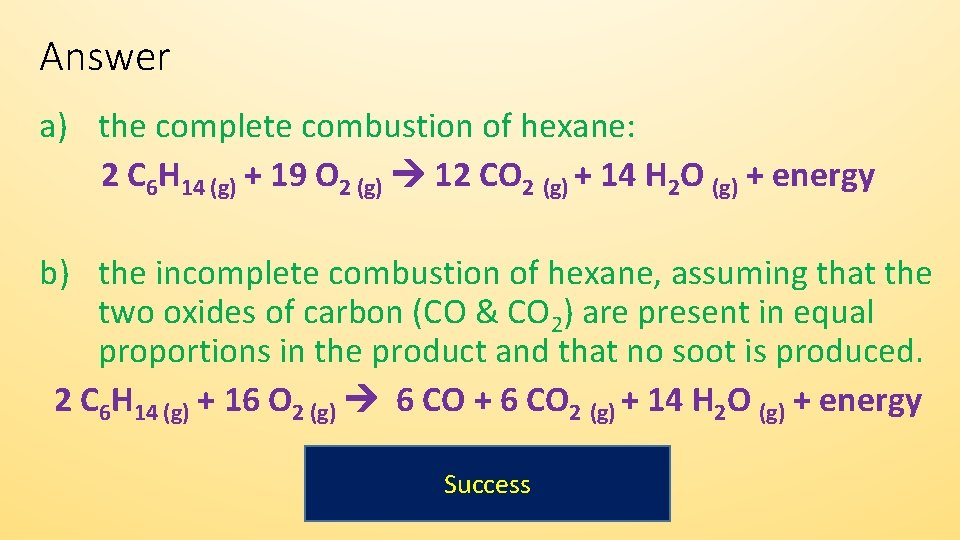Answer a) the complete combustion of hexane: 2 C 6 H 14 (g) + 19 O 2 (g) 12 CO 2 (g) + 14 H 2 O (g) + energy b) the incomplete combustion of hexane, assuming that the two oxides of carbon (CO & CO 2) are present in equal proportions in the product and that no soot is produced. 2 C 6 H 14 (g) + 16 O 2 (g) 6 CO + 6 CO 2 (g) + 14 H 2 O (g) + energy SuccessSuccess! You have reached the end of this activity. You will know that you have achieved the goals for this activity when you can describe and identify complete and incomplete combustion reactions, can give examples of these reactions and can predict the products of these reactions. Back to Start